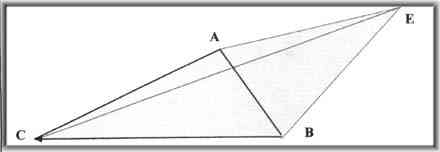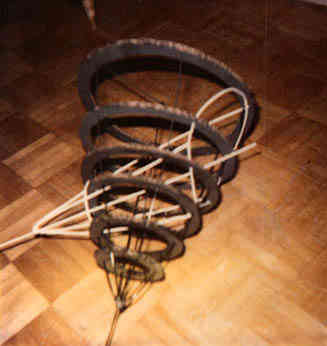THREE OBSERVERS SEE EVENT HORIZON FROM ONE EVENT The reader should be advised that the inclusion of 3 observers has been done as it represents the minimal amount of observers for fixed-point determinations. It is, however, more difficult to explain and probably to understand as well. To better grasp underlying concepts of fixed-point determinations, it is suggested that it may be easier to read the section on 4 observers first. In essence, this section is superfluous.A, B, and C are the fixed-point locations at their individual receipt times of observation. Triangle ABC would not be an equilateral triangle… most likely to be of ALL different lengths; (AB, AC, AD) at t = 0, let the mathematical Event E occur at mathematical point E having the coordinates EXYZ also at t = 0, are 4 points, that have been synchronized in time and are fixed in place in the shape of a regular tetrahedron. Points A, B, C, D at t = 0, each have coordinates, however at t = 0, they are completely irrelevant. ( t = 0 comes before t = 1 and then t = 2, etc.) at t = 1, The event itself, of course, is now over. at t = 1 point A receives the physical horizon from the event E in the matter form of photons. at t = 2, point B receives the horizon from the event E. at t = 3, point C observes photons arriving from event E. It is the intention of this portion to show that there is an absolute sequence of events. It is also written in an attempt to show a precise method for that determination of an absolute event sequence. To begin, it is perhaps best to discuss a single event and its perception. A single event, once it has occurred, will generate a spherical light horizon and with increasing diameter as the time interval increases from the point of the event. This perception of that event can only occur at the location of this spherical shell and is completely determinable should the velocity of the ever increasing radius be known, which in this case, is light. If two points of known distance from one another were to share a common time frame, (as synchronized as possible), then with a knowledge of the time interval as received at the two points, a possible event horizon can be generated. From the time that a particular event occurs, any two points that experience the light horizon from the event, and also share a common time and coordination frame reference with each other, can establish possible event locations. These possible event locations appear in the form of a parabolic shell bending back towards the closer of the two receiving locations. The shape of which is a 3-d parabolic shell called a paraboloid. This paraboloid will curve back around the initial's viewer's position; for the special case when both receive it at the same instant, the possible event location can only be from the vertical plane that is equidistant from both fixed-point locations at their own individual moment of receipt. When a third observer is added to the original two, then it is possible to generate an absolute time, (in reference to the three observers), and absolute location, (in reference to the three observers). If we begin with the paraboloid as established by observer 1 and 2, we can see that there are similar paraboloid that exist for observers 1 and 3, as well as a paraboloid for observers 2 and 3.The intersection of two parabolic cones results in the white, hoop-like location as the only possible fixed points from which the event may have occurred.Mathematically, the intersections of three paraboloids will generate only two possible locations for the event occurrence. These two points will in fact be equidistant from the plane as established by the three observer positions. The final point determination is made by simply knowing the side of the plane from which the event occurred. There exists only one case for which the time interval knowledge will not be sufficient to determine the location of an event. This singular case occurs when all three observer positions receive the event horizon at the same moment in time. In this case, there is in effect, no paraboloid that intersect. Since each observers receipt time is identical, then a PLANE of possible event locations exists between any two observers. The intersection of these three planes established by the interval times, results in a LINE representing the possible event locations. This line is perpendicular to the plane as established by the three observer positions. It passes through this plane as established by the three observer positions, at the point that is equidistant from all three. Its final determination can be verified any other groupings of observers that share the coordinate and time standards. Of course, the actual placement of a particular event would require that the three receipt times must be compiled and then calculated and for any of the three observers to know the absoluteness of its time or location, would require that a message be send from the compiler back to the specific observer. This would mean that a centralized data-bank of important events could be cataloged. Knowledge of absolute locations, albeit only of events already having occurred, is of possible great potential use. If we in fact can determine exactly where and when something was, then we should also take a look at performing an experiment in the laboratory. All that is required is three fixed observer positions to one another. Or even take 10 different positions, ... use any three of them and the exact location and time of any event or sequence of any events and the perception times at all points is quite absolute and determinable. The experiment could include multiple events as well, and through this paraboloid intersection calculation procedure, would generate not only the absolute sequence of events, but also the exact times and locations of the event horizons that were generated from the events.
BACK